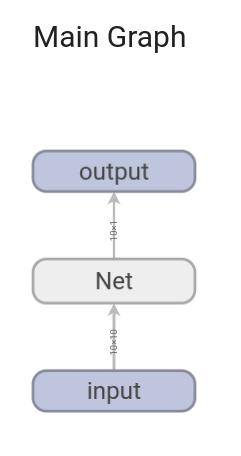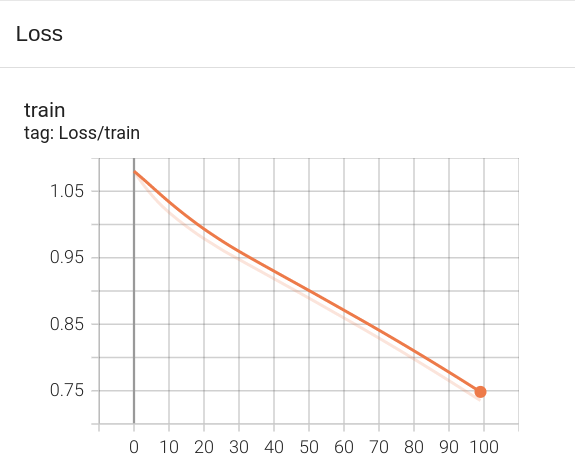# Visualizing PyTorch Models, Data, and Training with TensorBoard

TensorBoard is a visualization toolkit for machine learning experimentation. TensorBoard allows tracking and visualizing metrics such as loss and accuracy, visualizing the model graph, viewing histograms, displaying images, and much more. In this tutorial, we will learn how to use TensorBoard with PyTorch.

## Install TensorBoard

First, make sure that TensorBoard is installed. If not, you can install it with pip:

``````pip install tensorboard
``````

## Define a Simple Network

We’ll define a basic network for this example.

``````import torch
import torch.nn as nn
import torch.nn.functional as F
import torch.optim as optim
from torch.utils.tensorboard import SummaryWriter

class Net(nn.Module):
def __init__(self):
super(Net, self).__init__()
self.fc1 = nn.Linear(10, 20)
self.fc2 = nn.Linear(20, 1)

def forward(self, x):
x = F.relu(self.fc1(x))
x = self.fc2(x)
return x

net = Net()
``````

## Create a SummaryWriter

The SummaryWriter is your main entry to log data for consumption by TensorBoard.

``````writer = SummaryWriter('runs/experiment_1')
``````

## Writing to TensorBoard

Now let’s write a random input and the corresponding model graph to TensorBoard.

``````# Random input tensor
input_tensor = torch.rand(10, 10)

# Writing the model graph
``````

This will log the graph of the model and allow us to visualize it in TensorBoard.

## Running TensorBoard

To start TensorBoard and see the visualizations, we can run the following command in the terminal:

``````tensorboard --logdir=runs
``````

Then, open your web browser and go to `localhost:6006`. You should see the TensorBoard interface, where you can navigate to the “Graphs” tab to see the architecture of your model line below:## Logging Training Metrics

Let’s assume we have a simple training loop and we want to log the training loss. Here’s an example:

``````# Create a random target tensor
target = torch.randn(10, 1)

# Define a loss function and an optimizer
criterion = nn.MSELoss()
optimizer = optim.SGD(net.parameters(), lr=0.01)

for epoch in range(100):  # loop over the dataset multiple times

# forward + backward + optimize
output = net(input_tensor)
loss = criterion(output, target)
loss.backward()
optimizer.step()

# Write loss into the writer
In this example, we log the training loss at each epoch during training. After running this code, you can refresh TensorBoard, and you should see a “Scalars” tab where the training loss is plotted against the epoch number: